# Review — Exemplar-CNN: Discriminative Unsupervised Feature Learning with Convolutional Neural Networks (Self-Supervised Learning)

## Exemplar-CNN: Trained on Unlabeled Data Using Surrogate Class by Data Transformation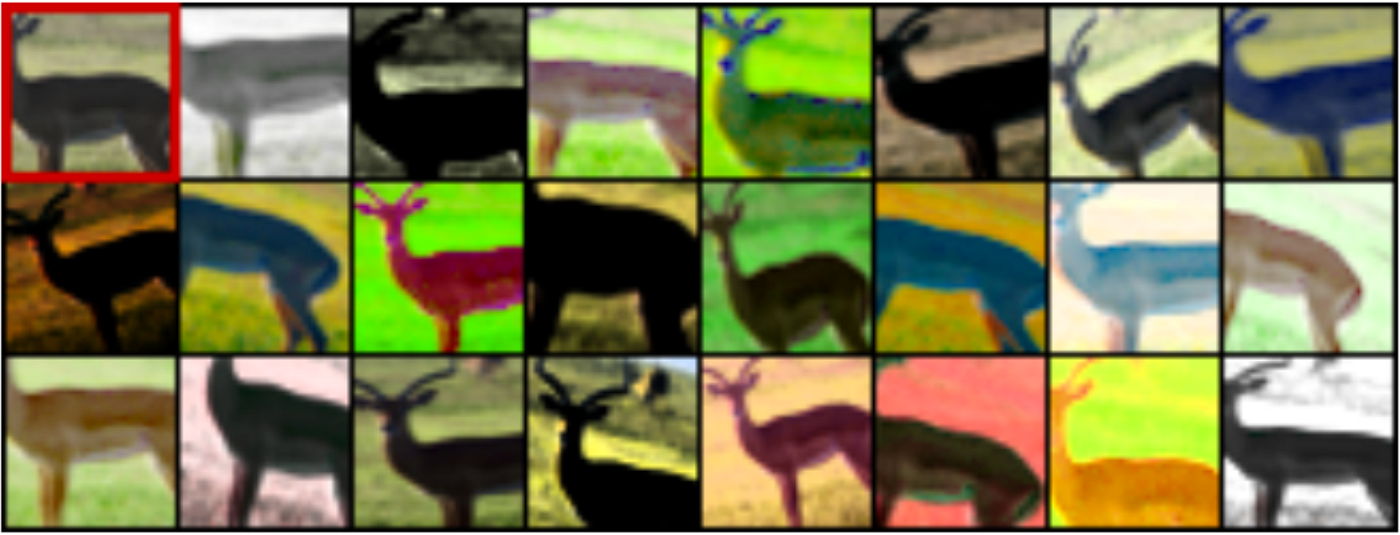Surrogate classes are generated by data transformation using unlabeled data

In this story, Discriminative Unsupervised Feature Learning with Convolutional Neural Networks, (Exemplar-CNN), by University of Freiburg, is reviewed. In this paper:

• Surrogate classes are generated using unlabeled data.
• Each surrogate class is formed by applying a variety of transformations to a randomly sampled “seed” image patch.
• CNN is trained to discriminate between a set of surrogate classes.

This is a paper in 2014 NIPS with over 600 citations. (Sik-Ho Tsang @ Medium)

# Outline

1. Creating Surrogate Training Data & Learning Algorithm
2. CNN Architectures & Experimental Setup
3. Experimental Results

# 1. Creating Surrogate Training Data & Learning Algorithm

Random transformation is applied to patches. All transformed patches from the same original “seed” image, are having the same surrogate class as the original “seed” images.

If there are 8000 “seed” images, then there are 8000 surrogate classes.

## 1.1. Creating Surrogate Training Data

• The input to the training procedure is a set of unlabeled images.
• N ∈ [50, 32000] patches of size 32×32 pixels are randomly sampled from different images at varying positions and scales forming the initial training set X = {x1, …, xN}.
• We are interested in patches containing objects or parts of objects, hence we sample only from regions containing considerable gradients.
• A family of transformations { | αA} is defined parameterized by vectors ∈ A, where A is the set of all possible parameter vectors. Each transformation T is a composition of elementary transformations from the following list:
1. Translation: vertical or horizontal translation by a distance within 0.2 of the patch size;
2. Scaling: the patch is scale by a factor between 0.7 and 1.4;
3. Rotation: rotation of the image by an angle up to 20 degrees;
4. Contrast 1: multiply the projection of each patch pixel onto the principal components of the set of all pixels by a factor between 0.5 and 2.
5. Contrast 2: raise saturation and value (S and V components of the HSV) of all pixels to a power between 0.25 and 4 (same for all pixels within a patch), multiply these values by a factor between 0.7 and 1.4, add to them a value between -0.1 and 0.1;
6. Color: add a value between -0.1 and 0.1 to the hue (H component of the HSV) of all pixels in the patch.
• For each initial patch xi X, K ∈ [1, 300] random parameter vectors {α1i, …, αKi} are sampled:
• And the corresponding transformations {T_α1i, …, T_αKi} to the patch xi. (i.e., to be brief, applying random transformation to each patch)
• This yields the set of its transformed versions Sxi = Tixi = {Txi | TTi}.
• Afterwards, the mean of each pixel over the whole resulting dataset are subtracted, and no any other preprocessing.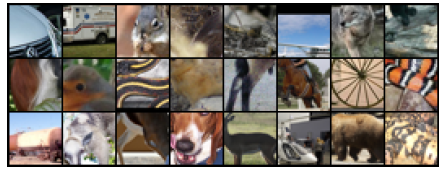Exemplary patches sampled from the STL unlabeled dataset which are later augmented by various transformations to obtain surrogate data for the CNN training.
• Exemplary patches sampled from the STL-10 unlabeled dataset are shown above.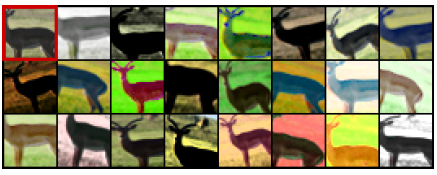Several random transformations applied to one of the patches extracted from the STL unlabeled dataset. The original (’seed’) patch is in the top left corner.
• Examples of transformed versions of one patch are shown above.

## 1.2. Learning Algorithm

With surrogated class generated, CNN can be trained.

• A CNN is trained to discriminate between these surrogate classes.
• Formally, we minimize the following loss function:
• Each of these sets to be a class by assigning label i to the class Sxi.
• where l(i, Txi) is the loss on the transformed sample Txi with (surrogate) true label i.

Intuitively, the classification problem described above serves to ensure that different input samples can be distinguished. At the same time, it enforces invariance to the specified transformations.

After training the CNN using unlabeled dataset, the CNN features are pooled are used to train a linear SVM for the target dataset, which will be mentioned in more details as below.

# 2. CNN Architectures & Experimental Setup

## 2.1. Unlabeled Dataset for Surrogate Class

• STL is especially well suited for unsupervised learning as it contains a large set of 100,000 unlabeled samples.
• Surrogate training data is extracted from unlabeled subset of STL-10.

## 2.2. Two CNNs

• Two networks are used: One is small and one is big.
• A “small” network: consists of two convolutional layers with 64 filters each followed by a fully connected layer with 128 neurons.
• A “large” network: consists of three convolutional layers with 64, 128 and 256 filters respectively followed by a fully connected layer with 512 neurons.
• All convolution is 5×5 filters. 2×2 max pooling is used after the first and second convolutions. Dropout is applied to fully connected layer.

## 2.3. Pooled Features for Linear SVM

• For STL-10 and CIFAR-10, to each feature map, 4-quadrant max-pooling, resulting in 4 values per feature map, is used.
• For Caltech-101, 3-layer spatial pyramid, i.e. max-pooling over the whole image as well as within 4 quadrants and within the cells of a 4×4 grid, resulting in 1 + 4 + 16 = 21 values per feature map, is used.
• A linear support vector machine (SVM) is trained on the pooled features.

# 3. Experimental Results

## 3.1. SOTA Comparison

The features extracted from the larger network match or outperform the best prior result on all datasets.

• This is despite the fact that the dimensionality of the feature vector is smaller than that of most other approaches and that the networks are trained on the STL-10 unlabeled dataset (i.e. they are used in a transfer learning manner when applied to CIFAR-10 and Caltech 101).

## 3.2. Number of Surrogate Classes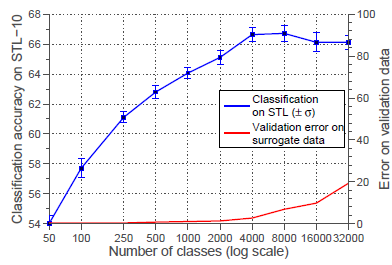Influence of the number of surrogate training classes
• The number N of surrogate classes is varied between 50 and 32000.

The classification accuracy increases with the number of surrogate classes until it reaches an optimum at about 8000 surrogate classes after which it did not change or even decreased.

• This is to be expected: the larger the number of surrogate classes, the more likely it is to draw very similar or even identical samples, which are hard or impossible to discriminate.
• This also demonstrates the main limitation of our approach to randomly sample “seed” patches: it does not scale to arbitrarily large amounts of unlabeled data.

## 3.3. Number of Samples per Surrogate Class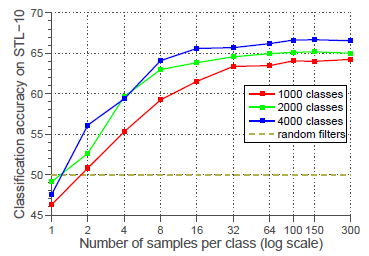Classification performance on STL for different numbers of samples per class
• The classification accuracy is shown when the number K of training samples per surrogate class varies between 1 and 300.
• As seen, if the number of samples is too small, there is insufficient data to learn the desired invariance properties.

The performance improves with more samples per surrogate class and saturates at around 100 samples.

## 3.4. Types of Transformations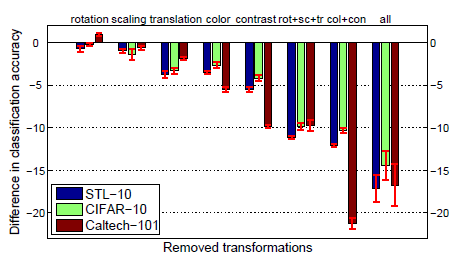Influence of removing groups of transformations during generation of the surrogate training data.
• The value “0” corresponds to applying random compositions of all elementary transformations: scaling, rotation, translation, color variation, and contrast variation.
• Different columns of the plot show the difference in classification accuracy as we discarded some types of elementary transformations.
• First, rotation and scaling have only a minor impact on the performance, while translations, color variations and contrast variations are significantly more important.
• Secondly, the results on STL-10 and CIFAR-10 consistently show that spatial invariance and color-contrast invariance are approximately of equal importance for the classification performance.
• Thirdly, on Caltech-101, color and contrast transformations are much more important compared to spatial transformations than on the two other datasets, since Caltech-101 images are often well aligned, and this dataset bias makes spatial invariance less useful.

## Reference

[2014 NIPS] [Exemplar-CNN]
Discriminative Unsupervised Feature Learning with Convolutional Neural Networks

## Self-Supervised Learning

2014 [Exemplar-CNN] 2015 [Context Prediction]

--

--